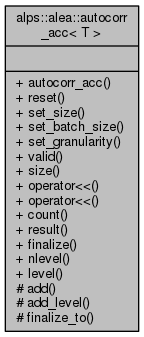ALPSCore reference
alps::alea::autocorr_acc< T > Class Template Reference

#include <autocorr.hpp>

Collaboration diagram for alps::alea::autocorr_acc< T >:## Public Types

using value_type = T

using var_type = typename bind< circular_var, T >::var_type

using level_acc_type = var_acc< T, circular_var >

## Public Member Functions

autocorr_acc (size_t size=1, size_t batch_size=1, size_t granularity=2)

void reset ()

void set_size (size_t size)

void set_batch_size (size_t batch_size)

void set_granularity (size_t granularity)

bool valid () const

size_t size () const

autocorr_accoperator<< (const computed< T > &src)

autocorr_accoperator<< (const autocorr_result< T > &result)

size_t count () const

autocorr_result< T > result () const

autocorr_result< T > finalize ()

size_t nlevel () const

const level_acc_typelevel (size_t i) const

## Protected Member Functions

void add (const computed< T > &source, size_t count)

void finalize_to (autocorr_result< T > &result)

## Friends

class batch_result< T >

## Detailed Description

### template<typename T> class alps::alea::autocorr_acc< T >

Accumulator for the integrated autocorrelation time.

The integrated autocorrelation time tau_int of a time series can be defined as the large-n limit of:

              1 + 2 * tau_int = var(n) / var(1),                    (A)


where var(n) is the sample variance obtained when averaging over batches, each batch being the sum of n consecutive elements of the series. Given a simulation of N steps, its corresponding squared error of the mean sq_error must thus be corrected as:

           sq_error = (1 + 2 * tau_int) * var(1) / N                (B)


which can be seen as replacing N with the number of uncorrelated samples. For a finite simulation, a tradeoff must be made between

(1) formal validity of above equations, which improves with n, (2) statistical uncertainty in tau_int, which improves with N/n.

This can be seen by plugging (A) into (B), which just yields the normal error estimate when sampling over bins of size n.

The class builds up a hierarchy of variance estimates for different batch sizes, starting with n=batch_size at level 0, and increasing by a factor granularity at each level. Assuming k-sized vectors, the estimator scales as O(k * log N) in memory and O(k * N * log log N) in runtime.

Definition at line 17 of file autocorr.hpp.

## Member Typedef Documentation

template<typename T >
 using alps::alea::autocorr_acc< T >::level_acc_type = var_acc

Definition at line 71 of file autocorr.hpp.

template<typename T >
 using alps::alea::autocorr_acc< T >::value_type = T

Definition at line 69 of file autocorr.hpp.

template<typename T >
 using alps::alea::autocorr_acc< T >::var_type = typename bind::var_type

Definition at line 70 of file autocorr.hpp.

## Constructor & Destructor Documentation

template<typename T >
 alps::alea::autocorr_acc< T >::autocorr_acc ( size_t size = 1, size_t batch_size = 1, size_t granularity = 2 )

Definition at line 15 of file autocorr.cpp.

## Member Function Documentation

template<typename T >
 void alps::alea::autocorr_acc< T >::add ( const computed< T > & source, size_t count )
protected

Definition at line 67 of file autocorr.cpp.

template<typename T >
 void alps::alea::autocorr_acc< T >::add_level ( )
protected

Definition at line 59 of file autocorr.cpp.

template<typename T >
 size_t alps::alea::autocorr_acc< T >::count ( ) const
inline

Returns sample size, i.e., number of accumulated data points

Definition at line 101 of file autocorr.hpp.

template<typename T >
 autocorr_result< T > alps::alea::autocorr_acc< T >::finalize ( )

Frees data associated with accumulator and return result

Definition at line 108 of file autocorr.cpp.

template<typename T >
 void alps::alea::autocorr_acc< T >::finalize_to ( autocorr_result< T > & result )
protected

Definition at line 116 of file autocorr.cpp.

template<typename T >
 const level_acc_type& alps::alea::autocorr_acc< T >::level ( size_t i ) const
inline

Definition at line 111 of file autocorr.hpp.

template<typename T >
 size_t alps::alea::autocorr_acc< T >::nlevel ( ) const
inline

Definition at line 109 of file autocorr.hpp.

template<typename T >
 autocorr_acc& alps::alea::autocorr_acc< T >::operator<< ( const computed< T > & src )
inline

Add computed vector to the accumulator

Definition at line 95 of file autocorr.hpp.

template<typename T >
 autocorr_acc< T > & alps::alea::autocorr_acc< T >::operator<< ( const autocorr_result< T > & result )

Merge partial result into accumulator

Definition at line 82 of file autocorr.cpp.

template<typename T >
 void alps::alea::autocorr_acc< T >::reset ( )

Re-allocate and thus clear all accumulated data

Definition at line 27 of file autocorr.cpp.

template<typename T >
 autocorr_result< T > alps::alea::autocorr_acc< T >::result ( ) const

Returns result corresponding to current state of accumulator

Definition at line 99 of file autocorr.cpp.

template<typename T >
 void alps::alea::autocorr_acc< T >::set_batch_size ( size_t batch_size )

Update the batch size and discard all measurements

Definition at line 43 of file autocorr.cpp.

template<typename T >
 void alps::alea::autocorr_acc< T >::set_granularity ( size_t granularity )

Update the increment between levels and discard all measurements

Definition at line 51 of file autocorr.cpp.

template<typename T >
 void alps::alea::autocorr_acc< T >::set_size ( size_t size )

Update the size and discard all measurements

Definition at line 36 of file autocorr.cpp.

template<typename T >
 size_t alps::alea::autocorr_acc< T >::size ( ) const
inline

Number of components of the random vector (e.g., size of mean)

Definition at line 92 of file autocorr.hpp.

template<typename T >
 bool alps::alea::autocorr_acc< T >::valid ( ) const
inline

Returns false if finalize() has been called, true otherwise

Definition at line 89 of file autocorr.hpp.

## Friends And Related Function Documentation

template<typename T >
 friend class batch_result< T >
friend

Definition at line 124 of file autocorr.hpp.

The documentation for this class was generated from the following files: